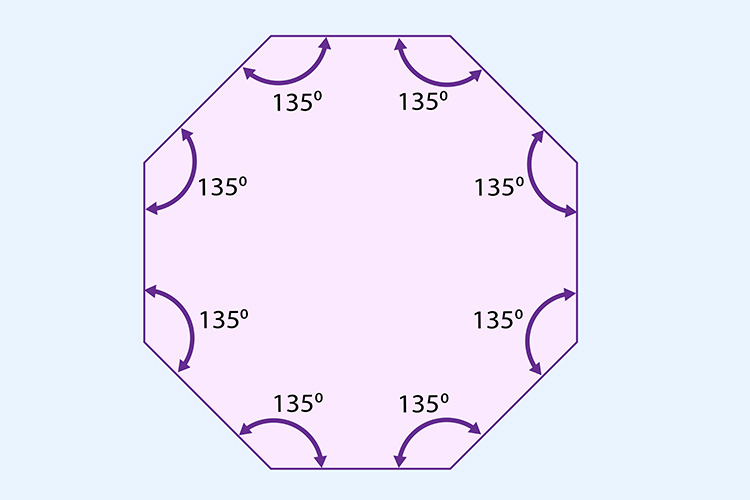# The total interior angles of an octagon = 1080°

Each time we add a side (triangle to square, square to pentagon, pentagon to hexagon) we add another 180°.

## Triangle to octagon

 Triangle Square Pentagon Hexagon Heptagon Octagon 180° + 180° + 180° + 180° + 180° + 180° = 1080°

The total internal angles of a heptagon = 1080°Now, divide the total internal angle by the number of corners.

i.e. divide 1080° by 8.

1080^circ/8=135^circ

 1 2 3 4 5 6 7 8 135° + 135° + 135° + 135° + 135° + 135° + 135° + 135° = 1080°